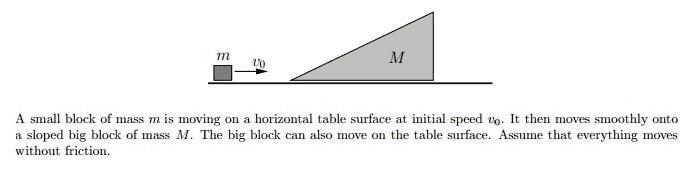# Conservation of Momentum/Mechanical Energy Question (Fairly Challenging)

Quotexon

## Homework Statement

A small block of mass m is moving on a horizontal table surface at initial speed v0. It then moves smoothly onto a sloped big block of mass M. The big block can also move on the table surface. Assume that everything moves without frictionFind the speed v of the small block after it leaves the slope.

## Homework Equations

Conservation of Momentum: mv0 = (M+m)v → v=(mv0)/(M+m)
Conservation of Mechanical Energy: fairly obvious
The height that it rises to is h = (1/2g)(Mv0^2/M+m), I derived this from conservation of momentum and conservation of mechanical energy

## The Attempt at a Solution

I set up the conservation of momentum: (M + m)v = -mv1 + Mv2 Is this correct?
Now I ask, how should I set up the conservation of mechanical energy equation? Should I solve for one variable in terms of the other? The answer should be in terms of v0.
The answer is v1 = ((M-m)/(M+m))v0

Last edited:

## Answers and Replies

Mentor
Conservation of Momentum: mv0 = (M+m)v → v=(mv0)/(M+m)
Don't assume that m & M have the same velocity.
Conservation of Mechanical Energy: fairly obvious
The height that it rises to is h = (1/2g)(Mv0^2/M+m), I derived this from conservation of momentum and conservation of mechanical energy
Don't worry about the height. That's an intermediate point that we don't care about.

## The Attempt at a Solution

I set up the conservation of momentum: (M + m)v = -mv1 + Mv2 Is this correct?
Set initial momentum equal to final momentum. Let v1 and v2 be the final velocities after they separate.
Now I ask, how should I set up the conservation of mechanical energy equation?
Intial KE = final KE
Should I solve for one variable in terms of the other? The answer should be in terms of v0.
You'll have two equations. Eliminate v2 and solve for v1.

Quotexon
How would a conservation of kinetic energy equation look like?

1/2(M+m)v^2 = 1/2mv1^2 + 1/2Mv2^2 ?

Conservation of momentum: (M+m)v = -mv1 + Mv2 ?

If I solve for v1 using these equations, it turns out very convoluted and does not resemble the answer. I feel as though it should be cleaner than this. Am I doing something incorrectly?

Quotexon
Does this problem even meet the criteria to use conservation of kinetic energy? It doesn't seem to be elastic, does it?

Please help, this problem has been bugging me for awhile.

Mentor
How would a conservation of kinetic energy equation look like?

1/2(M+m)v^2 = 1/2mv1^2 + 1/2Mv2^2 ?
OK, except for the left hand side. Initially, only m is moving.

Conservation of momentum: (M+m)v = -mv1 + Mv2 ?
Same issue as above.

If I solve for v1 using these equations, it turns out very convoluted and does not resemble the answer. I feel as though it should be cleaner than this. Am I doing something incorrectly?
Clean up the equations as I suggest and try again. A bit of a pain, but you'll get the required answer with a bit of work.

Quotexon
Wow, it worked! Thanks so much for your help. I greatly appreciate it.

Mentor
Excellent!(And you are most welcome.)